## RS Aggarwal Class 7 Solutions Chapter 2 Fractions Ex 2C

These Solutions are part of RS Aggarwal Solutions Class 7. Here we have given RS Aggarwal Solutions Class 7 Chapter 2 Fractions Ex 2C.

Other Exercises

Question 1.
Solution: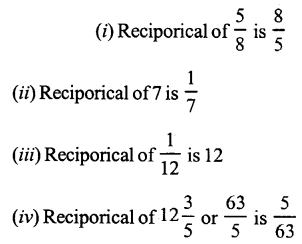Question 2.
Solution: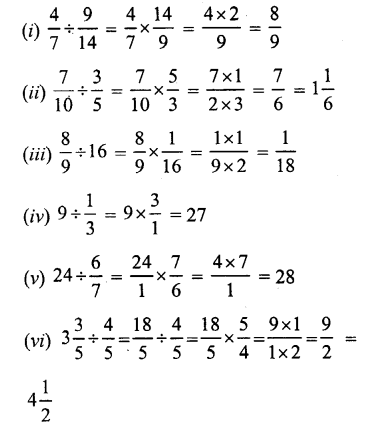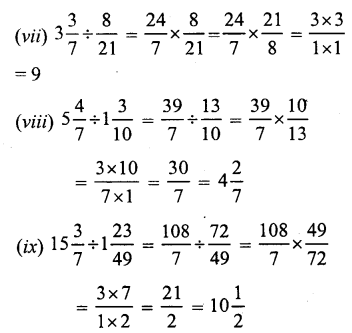Question 3.
Solution: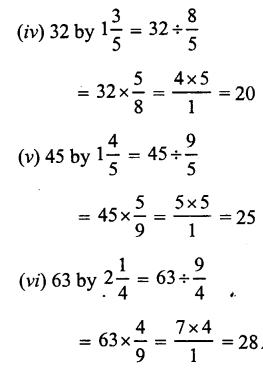Question 4.
Solution: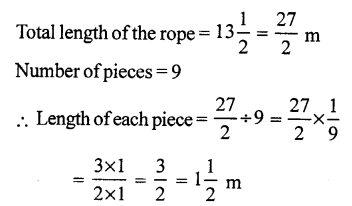Question 5.
Solution:
Total weight of 18 boxes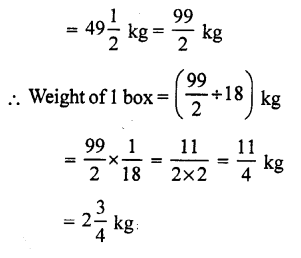Question 6.
Solution:
Total amount of oranges=Rs 378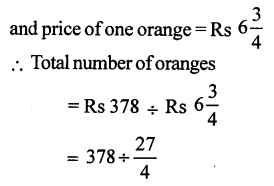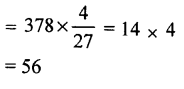Question 7.
Solution: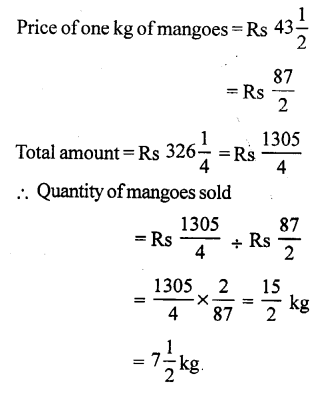Question 8.
Solution:Question 9.
Solution: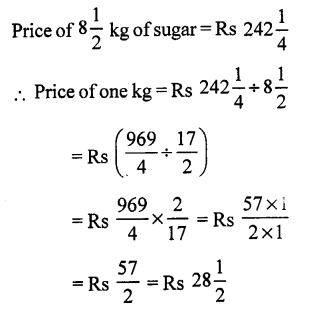Question 10.
Solution: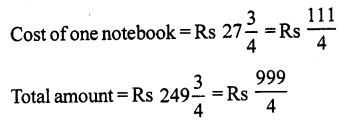Question 11.
Solution: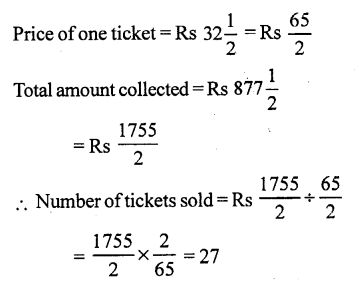Question 12.
Solution: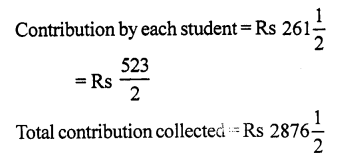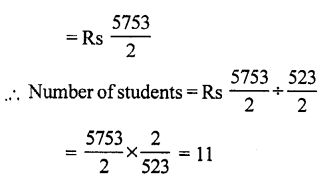Question 13.
Solution:
Total quantity of milk = 24 litres
and quantity of milk got by one student = $$\frac { 2 }{ 5 }$$ litresQuestion 14.
Solution:
Quantity of water in a bucket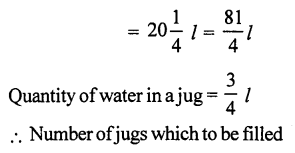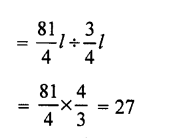Question 15.
Solution: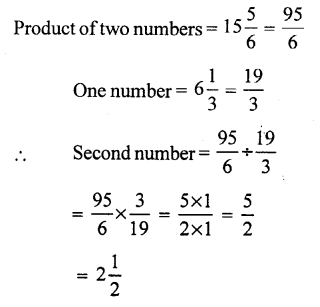Question 16.
Solution:
Product of two numbers = 42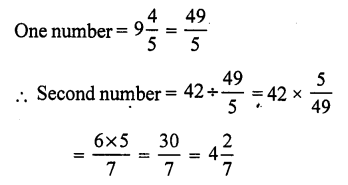Question 17.
Solution:Hope given RS Aggarwal Solutions Class 7 Chapter 2 Fractions Ex 2C are helpful to complete your math homework.

If you have any doubts, please comment below. Learn Insta try to provide online math tutoring for you.